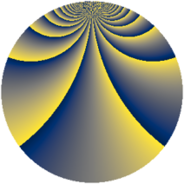# Properties

 Label 1815.2.bnLevel $1815$ Weight $2$ Character orbit 1815.bn Rep. character $\chi_{1815}(29,\cdot)$ Character field $\Q(\zeta_{110})$ Dimension $10400$ Sturm bound $528$

# Related objects

## Defining parameters

 Level: $$N$$ $$=$$ $$1815 = 3 \cdot 5 \cdot 11^{2}$$ Weight: $$k$$ $$=$$ $$2$$ Character orbit: $$[\chi]$$ $$=$$ 1815.bn (of order $$110$$ and degree $$40$$) Character conductor: $$\operatorname{cond}(\chi)$$ $$=$$ $$1815$$ Character field: $$\Q(\zeta_{110})$$ Sturm bound: $$528$$

## Dimensions

The following table gives the dimensions of various subspaces of $$M_{2}(1815, [\chi])$$.

Total New Old
Modular forms 10720 10720 0
Cusp forms 10400 10400 0
Eisenstein series 320 320 0

## Trace form

 $$10400q - 420q^{4} - 34q^{6} - 54q^{9} + O(q^{10})$$ $$10400q - 420q^{4} - 34q^{6} - 54q^{9} - 66q^{10} - 14q^{15} + 124q^{16} - 156q^{19} - 154q^{21} - 80q^{24} - 88q^{25} - 87q^{30} - 144q^{31} - 120q^{34} - 10q^{36} - 104q^{39} - 170q^{40} - 43q^{45} - 136q^{46} + 36q^{49} - 96q^{51} - 198q^{54} - 180q^{55} - 51q^{60} - 176q^{61} - 536q^{64} - 112q^{66} - 22q^{69} - 26q^{70} - 82q^{75} - 176q^{76} - 132q^{79} + 10q^{81} - 294q^{84} - 58q^{85} - 65q^{90} - 76q^{91} - 280q^{94} + 48q^{96} - 346q^{99} + O(q^{100})$$

## Decomposition of $$S_{2}^{\mathrm{new}}(1815, [\chi])$$ into newform subspaces

The newforms in this space have not yet been added to the LMFDB.